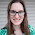Math = Love: Adding and Subtracting Polynomials in Function Notation Question Stack

## Thursday, February 23, 2017

### Adding and Subtracting Polynomials in Function Notation Question Stack

My Algebra 1 students are currently working their way through our unit on polynomials.  We have new math standards in Oklahoma this year which has required me to change things up a bit.  In the past, my students needed to know how to add, subtract, multiply, and factor polynomials.  The new standards STILL have them doing all of that.  But, they also need to be able to add, subtract, and multiply functions written in function notation.  We do functions and function notation waaaaaaaaaaaayyyyyy before we ever talk about polynomials.  So, I decided to kill two birds with one stone and combine these two skills.

I made a question stack for my students to use to practice adding and subtracting polynomials in function notation.  If you don't know how a question stack works, this post explains it pretty well.  Basically, students are given a set of 10 double-sided cards.  Students lay the cards out with the ANSWERS facing up to form an answer bank.  Students choose one card to flip over.  This will be the first question.  When they figure out the answer, they locate that answer in the answer bank.  That card is flipped over and stacked on top of the previous card to reveal a new question.  The answer bank gets smaller and smaller as students work problem after problem.  It's self-checking which means I can target my time to helping the groups who need it most.

Each group got a laminated page featuring four polynomials and a snack bag with their deck of cards in it.

The four polynomials:

Here are the 10 problems students worked through as they completed the question stack:

I tried my best to vary the notation so students would be used to the different ways the question could be worded.

Here is the answer bank:

I had my students work in pairs for this activity.  In some class periods, a few students would choose to work alone.  I also had the occasional group of three when the class consisted of an odd number of students.

Action pics:

My students were engaged.  There was good group-talk going on.  And, we did lots of practice.

Files for this activity are uploaded here.

1.1.